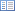Code of Federal Regulations (Last Updated: April 25, 2019)Title 40 - Protection of EnvironmentCHAPTER I — ENVIRONMENTAL PROTECTION AGENCYSUBCHAPTER C — AIR PROGRAMSPart 60 - STANDARDS OF PERFORMANCE FOR NEW STATIONARY SOURCESSubpart AAAA — Standards of Performance for Small Municipal Waste Combustion Units for Which Construction is Commenced After August 30, 1999 or for Which Modification or Reconstruction is Commenced After June 6, 2001

# § 60.1460 What equations must I use?

• (a) Concentration correction to 7 percent oxygen. Correct any pollutant concentration to 7 percent oxygen using equation 1 of this section:

C7% = Cunc * (13.9) * (1/(20.9−CO2)) (Eq.1)

(b) Percent reduction in potential mercury emissions. Calculate the percent reduction in potential mercury emissions (%PHg) using equation 2 of this section:

%PHg = (Ei−o) * (100/Ei) (Eq. 2)

(c) Percent reduction in potential hydrogen chloride emissions. Calculate the percent reduction in potential hydrogen chloride emissions (%PHC1) using equation 3 of this section:

%PHC1 = (Ei−o) * (100/Ei) (Eq. 3)

(d) Capacity of a municipal waste combustion unit. For a municipal waste combustion unit that can operate continuously for 24-hour periods, calculate the municipal waste combustion unit capacity based on 24 hours of operation at the maximum charge rate. To determine the maximum charge rate, use one of two methods:

(1) For municipal waste combustion units with a design based on heat input capacity, calculate the maximum charging rate based on the maximum heat input capacity and one of two heating values:

(i) If your municipal waste combustion unit combusts refuse-derived fuel, use a heating value of 12,800 kilojoules per kilogram (5,500 British thermal units per pound).

(ii) If your municipal waste combustion unit combusts municipal solid waste, use a heating value of 10,500 kilojoules per kilogram (4,500 British thermal units per pound).

(2) For municipal waste combustion units with a design not based on heat input capacity, use the maximum designed charging rate.

(e) Capacity of a batch municipal waste combustion unit. Calculate the capacity of a batch municipal waste combustion unit as the maximum design amount of municipal solid waste they can charge per batch multiplied by the maximum number of batches they can process in 24 hours. Calculate the maximum number of batches by dividing 24 by the number of hours needed to process one batch. Retain fractional batches in the calculation. For example, if one batch requires 16 hours, the municipal waste combustion unit can combust 24/16, or 1.5 batches, in 24 hours.

(f) Quarterly carbon usage. If you use activated carbon to comply with the dioxins/furans or mercury limits, calculate the required quarterly usage of carbon using equation 4 of this section for plant basis or equation 5 of this section for unit basis:

(1) Plant basis.